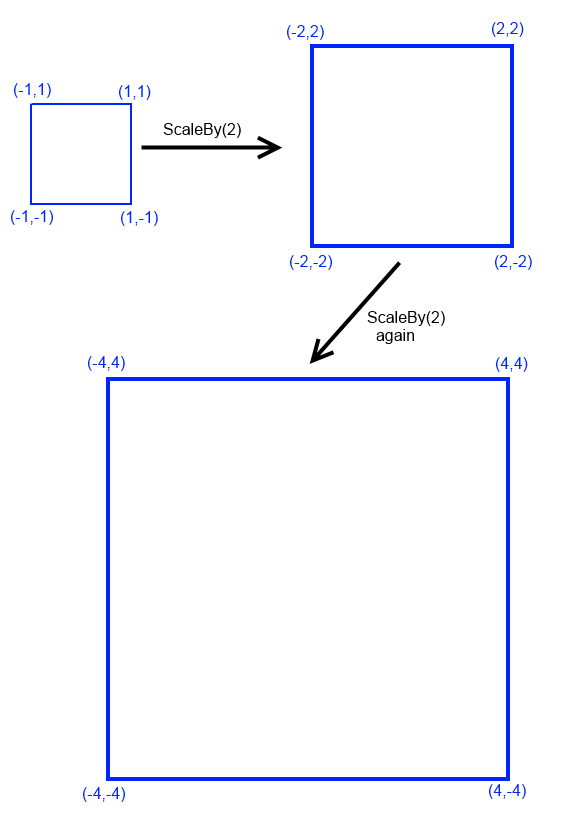# FlatRedBall.Math.Geometry.Polygon.ScaleBy

## Introduction

The ScaleBy method can be used to adjust a Polygon’s points to make it larger or smaller. The ScaleBy method is relative to the current state of the polygon. This means that calling ScaleBy with any number other than 1 multiple times will continually change the polygon.## Scaling multiple times

Calling ScaleBy multiple times will continually change the polygon. For example:

```myPolygon.ScaleBy(2);
```

will double the relative value of each point. Calling the method again will double it once more.

Ignoring floating point inaccuracy, the following line of code will result in no changes to a Polygon:

```myPolygon.ScaleBy(2);
myPolygon.ScaleBy(.5f);
```

## Preserving original shape

Since ScaleBy modifies the points on a Polygon, the Polygon has no built-in way to preserve the original shape of the polygon. To do so, you will have to keep track of that information yourself:

```// Assuming PolygonInstance is a valid Polygon:
float scaleAmount = 1;
PolygonInstance.ScaleBy(2);
scaleAmount *= 2;

PolygonInstance.ScaleBy(4);
scaleAmount *= 4;

// Now let's bring it back to its original shape:
PolygonInstance.ScaleBy( 1 / scaleAmount);
scaleAmount = 1;
```